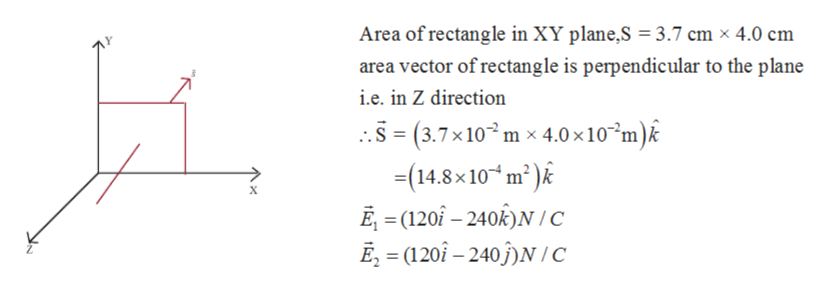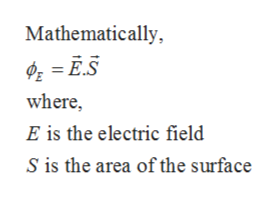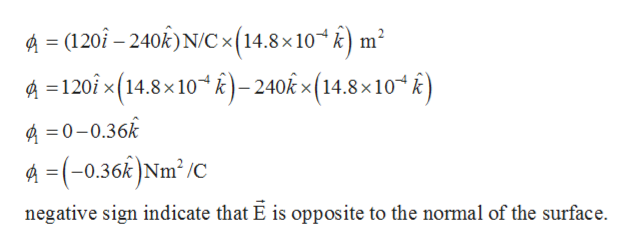# A 3.7 cm × 4.0 cm rectangle lies in the xy-plane. What is the electric flux through the rectangle if E (vector) =(120ı^−240k^)N/C? What is the electric flux through the rectangle if E (vector) =(120ı^−240ȷ^)N/C?

Question
107 views

A 3.7 cm × 4.0 cm rectangle lies in the xy-plane.

What is the electric flux through the rectangle if E (vector) =(120ı^−240k^)N/C?

What is the electric flux through the rectangle if E (vector) =(120ı^−240ȷ^)N/C?

check_circle

star
star
star
star
star
1 Rating
Step 1

Given:help_outlineImage TranscriptioncloseArea of rectangle in XY plane,S 3.7 cm x 4.0 cm area vector of rectangle is perpendicular to the plane i.e. in Z direction ..3(3.7x10 m x 4.0x 10~m)k -14.8x 10* m2)k х E =(120f -240k)N/c E = (120i- 240)N/c fullscreen
Step 2

Electric flux is defined as the number of electric field lines passing through the surface perpendicular to it.help_outlineImage TranscriptioncloseMathematically E.S where E is the electric field S is the area of the surface fullscreen
Step 3

Electric flux for 1st part...help_outlineImage Transcriptionclose= (120i-240k) N/C x(14.8 x 10) m A=120ix(14.8x 10*k)-240kx(14.8x10" k) 0-0.36k A (-0.36kNm2 /C negative sign indicate that E is opposite to the normal of the surface. fullscreen

### Want to see the full answer?

See Solution

#### Want to see this answer and more?

Solutions are written by subject experts who are available 24/7. Questions are typically answered within 1 hour.*

See Solution
*Response times may vary by subject and question.
Tagged in

### Physics